﻿ Predict Wins and Losses with Sci-kit Learn Decision Trees and SMS - TwilioLevel up your Twilio API skills in TwilioQuest, an educational game for Mac, Windows, and Linux. Download Now

# Predict Wins and Losses with Sci-kit Learn Decision Trees and SMS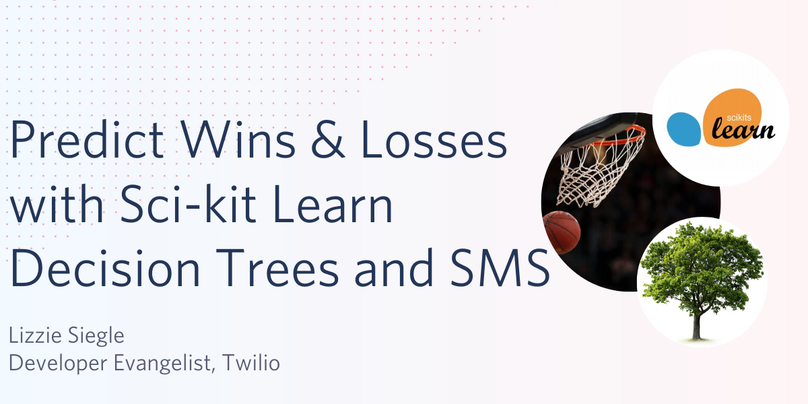I've been missing professional sports which are canceled for the time being. It got me thinking -- can we predict a basketball game outcome with machine learning? What stats of an individual player would best predict a team's win or a loss? This post will try to answer those questions (using Klay Thompson stats) with Pandas and sci-kit learn based on user input via a Twilio SMS containing a certain basketball statistic. With SMS user input, it is easy to compare which statistics are better suited for predicting whether the team won or loss because of that individual player.## Soo...Decision Trees

They make a training model to predict the outcome, class, or value of a target variable based on rules the model learns from input data. They model decisions in a tree-like manner drawn upside-down with the root at the top. Below is a weather decision tree from Juniata College deducing whether it is sunny, overcast, or raining.Decision trees are often used for both classification (output is categorical and discrete) and regression (result is numerical and continuous) in machine learning. Decision tree algorithms are sometimes called CART (Classification and Regression Trees).

Some vocabulary often used when talking about decision trees:

1. Entropy, or the amount of variance in the data. If a bag of M&M's had only brown ones it would have low entropy; if a bag of M&M’s had brown, red, green, yellow, and orange, the entropy would be high.
2. Root node: the top of the tree and where the training data or sample begins before being split into two or more sets.
3. Split: the tree splits or branches off into child nodes based on an attribute.
4. Leaf or terminal node: a node that does not have child nodes.

In short, decision trees identify ways to split a data sample according to different conditions. They can predict future outcomes and assign probabilities to those outcomes, determine whether or not to continue an experiment, and more. This blog post will show you how to code decision trees with some popular data mining and machine learning Python libraries.

## Prerequisites

### Setup your Python Project and a Twilio Number

Activate a virtual environment in Python 3 and on the command line run the following to install our dependencies:

```pip3 install sklearn pandas numpy flask twilio
```

Your Flask app will need to be visible from the web so Twilio can send requests to it. Ngrok lets us do this. With it installed, run the following command in your terminal in the directory your code is in in a new terminal tab: `ngrok http 5000`.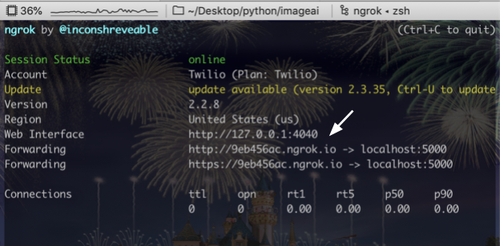You should see the screen above. Grab the https://  ngrok forwarding URL to configure your Twilio number in your phone number console. If you don't have a Twilio number yet, go to the Phone Numbers section of your Twilio Console and search for a phone number in your country and region, making sure the SMS checkbox is ticked.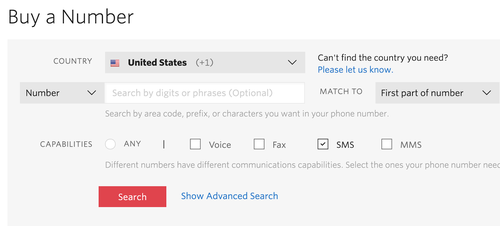In the `Messaging` section of your purchased number, in the `A Message Comes In`section, set the `Webhook` to be your ngrok https URL, appending `/sms` to the URL. Hit Save.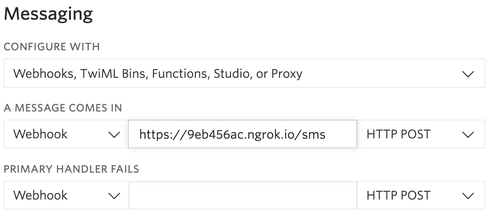## Access and Clean Data

As with most ML tasks, we need good data. I like the basketball team the Golden State Warriors, so I wanted data related to them. I found game-by-game data for individual players. Klay Thompson played quite a few games (and is one half of the Splash Brothers) so I used this game-by-game stats from him for the 2018-2019 season: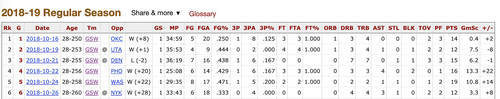If you are not interested in the process I used to export and clean up this data you can skip the rest of this section and use this file containing the clean data from GitHub.

1. Hover over Share & More
2. Click Modify & Share Table1. Select comma-separated under Share or get code for table as:
2. Copy-and-paste this data to a .csv file (my folder is called klay and the file klay_season_stats.csv).

Some of the columns have empty labels. Hand-edit the CSV file so that there is a H-A column header label (for home or away) at the top in-between Tm and Opp and a Win-Loss column header label in-between Opp and GS. Search and replace "W (" with "W", "L (" with "L", and ")" with ",". ("W" represents a win and "L" represents a loss.) After Win-Loss, add a header WLD that has the difference in scores which follow the Win-Loss. That should end up looking like this: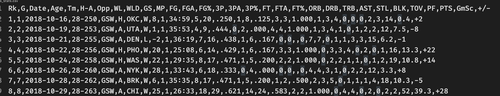The updated and cleaned data can be found here on GitHub.

## Read and Manipulate Data with Pandas

At the top of your Python file (mine is called `predict_with_klay.py`), import the required libraries.

```from sklearn.metrics import confusion_matrix, classification_report,  accuracy_score
from sklearn import tree, preprocessing
from sklearn.model_selection import train_test_split, cross_val_score
from sklearn.tree import DecisionTreeClassifier
import pandas as pd
import numpy as np
from twilio.twiml.messaging_response import MessagingResponse
```

Then save the csv file as a variable and set the columns we want to consider for this blog post.

```csv = 'klay_season_stats.csv'
cols = ['FG', 'FGA', 'FG%', '3P', '3PA', '3P%',
'FT', 'FTA', 'FT%', 'ORB', 'DRB', 'TRB']
```

Add the following code below to make a function that takes in the CSV file and an incoming SMS message that contains a statistic like FG, FGA, etc.

```def setup_df(file, msg):
print(df.shape) # (83, 31) = 83 records/rows, 31 attributes/columns
df = df[['H-A', 'FG%', '3P%', 'PTS', msg, 'WL']] #last item = classifier

#remove row with missing values
df = df.fillna(0)

#convert strings to integers
df['WL'] = df['WL'].map({'W': 1, 'L': 0})
df['H-A'] = df['H-A'].map({'H': 1, 'A': 0})

#access row with Did Not Dress, etc, replacing Did Not Dress, Inactive with 0's
#0 axis = rows, 1 axis = columns
m1 = df.eq('Did Not Dress').any(axis=1)
m2 = df.eq('Did Not Dress').any(axis=0)
m3 = df.eq('Inactive').any(axis=1)
m4 = df.eq('Inactive').any(axis=0)

df.loc[m1] = 0
df.loc[:, m2] = 0
df.loc[m3] = 0
df.loc[:, m4] = 0
return df
```

The function makes a Pandas data frame `df`, a 2D tabular data structure with labeled axes for rows and columns to make manipulating your data easier. Then we set df to be only the classes we want to examine (H-A, FG%, 3P%, PTS, and the user's input.) The last item in that array, whether the Warriors won or loss, is the classifier, or what we want to predict. We remove any rows with missing values, convert strings to integers: Any Win will be converted to 1 and Loss to 0. Then we look for games where Klay Thompson did not dress or was inactive. We still want to see whether or not the Warriors won with him gone, so we convert those values to zero. This isn't always the best method as NaN values could still have significance in being absent from the dataset. Introducing zeros could skew the data, but in this case zero is an acceptable value because Klay did have zero three-pointers, field goals, etc. in those games.

Now we clean the dataset of NaN, Inf, and missing cells for skewed datasets, keeping the DataFrame with valid entries in the same variable.

```def clean_dataset(df):
df.dropna(inplace=True)
indices_to_keep = ~df.isin([np.nan, np.inf, -np.inf]).any(1)
return df[indices_to_keep].astype(np.float64)
```

## Train, Make, Evaluate Predictions

First we need a Flask application to get the inbound text message. We define a `/sms` endpoint that listens to POST requests. We then check that the message is in the `cols` list of statistics we're considering. If so, we call the `setup_df` method to make our data frame data structure with the CSV file and inbound SMS before passing that `df` to `clean_dataset` to clean it into data we can use. A variable `X` holds every column from our dataset except the "WL" column, which is the label and a ` y` variable contains the classification class values from the "WL" column (whether the Warriors won or lost).

```app = Flask(__name__)
@app.route("/sms", methods=['GET', 'POST'])
def sms():
resp = MessagingResponse()
inb_msg = request.form['Body']
if inb_msg in cols:
df = setup_df(csv, inb_msg)
clean_dataset(df)
print(df.head())  # first 5 records of dataset
print(df)  # only data we're looking at with user input (also H-A, FG)
X = df.drop('WL', axis=1)
y = df['WL']
```

Then we use Scikit-Learn's `model_selection` library's `train_test_split` method to split the data into training and testing sets. The training set is used to check that the algorithm recognizes patterns in the data and the testing set is used to see how well the algorithm can predict new answers based on its training.

`test_size` sets the ratio of the test set used to split-up 20% of the data into the test set and 80% for the training set.

```        X_train, X_test, y_train, y_test = train_test_split(
X, y, test_size=0.20)
```

The `DecisionTreeClassifier` class provides an algorithm to train on the testing and training data and make predictions with. The `fit` method trains the algorithm on the training data passed as a parameter to it.

```        model = tree.DecisionTreeClassifier()
model.fit(X_train, y_train)
```

Lastly we make our predictions:

```        y_pred = model.predict(X_test)
```

Sci-kit Learn provides some handy-dandy methods to evaluate those predictions. classification_report provides precision (of the positive classes correctly predicted, the number that is actually positive), recall (number predicted correctly--the higher, the better), F1 (harmonic mean between precision and recall), and support score (number of occurrences of the given class in the dataset) for the model. The following images are when "ORB" for offensive rebounds are considered as a statistic.

```        print(classification_report(y_test, y_pred))
```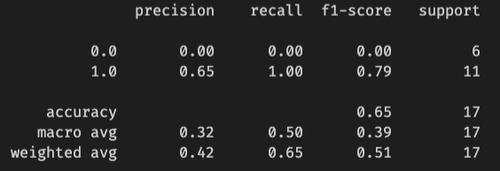Then the `cross_val_score` function performs cross-validation, testing the result by setting the `scoring` parameter to `accuracy`.

```        accuracy = np.mean(cross_val_score(model, X_test, y_test, scoring='accuracy')) * 100
print("Accuracy: {}%".format(accuracy))
```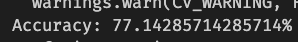Your accuracy will be different, but this accuracy is pretty good. Anything over 50% means the model is better than random. It means that the decision tree model has correctly classified 77% of the sample correctly as the Warriors winning or losing based on the user input. (Again, this accuracy includes offensive rebounds.)

Lastly, the Confusion Matrix returns the correct and incorrect classifications of our decision tree model, or the predicted outcomes compared to the actual outcomes.

```        print('confusion matrix {}'.format(pd.DataFrame(
confusion_matrix(y_test, y_pred),
columns=['Predicted Loss', 'Predicted Win'],
index=['True Loss', 'True Win']
)))
```

The confusion matrix returns four values without normalization: True Positive, True Negative, False Positive, and False Negative. They are in the locations below.

 True Positive False Positive False Negative True Negative

Our decision tree model returns no true positives and no false negatives, and four false positives and thirteen true negatives.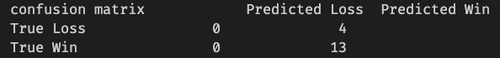We then return these performance metrics in an outbound message.

```        msg = 'The Decision Tree model correctly predicted if the Warriors won or lost based on some of Klay\'s stats {:.2f} % of the time.\n The confusion matrix looks like this:\n {}'.format(
accuracy, confusion_matrix(y_test, y_pred))
```

If the user does not send a statistic in our `cols` list, we tell them what messages they should send and then return our message.

```    else:
msg = "Send a message according to Klay Thompson's stats columns: {}".format(cols)
resp.message(msg)
return str(resp)
```

The complete code for that function is below.

```app = Flask(__name__)
@app.route("/sms", methods=['GET', 'POST'])
def sms():
resp = MessagingResponse()
inb_msg = request.form['Body']
if inb_msg in cols:
df = setup_df(csv, inb_msg)
clean_dataset(df)
print(df.head())  # first 5 records of dataset
print(df)  # only data we're looking at with user input (also H-A, FG)
X = df.drop('WL', axis=1)
y = df['WL']
# specifies ratio of test set, used to split up 20% of the data into test set and 80% for training
X_train, X_test, y_train, y_test = train_test_split(
X, y, test_size=0.20)  # random_state=1
model = tree.DecisionTreeClassifier()
# print(model)
model.fit(X_train, y_train)
y_pred = model.predict(X_test)
print(classification_report(y_test, y_pred))
accuracy = np.mean(cross_val_score(model, X_test, y_test, scoring='accuracy')) * 100
print("Accuracy: {}%".format(accuracy))

print('confusion matrix {}'.format(pd.DataFrame(
confusion_matrix(y_test, y_pred),
columns=['Predicted Loss', 'Predicted Win'],
index=['True Loss', 'True Win']
)))
# distributions for each group
msg = 'accuracy score: {}\n confusion matrix: {}\n distributions for predicted group: {}\n'.format(
accuracy, confusion_matrix(y_test, y_pred), y_test.value_counts(normalize=True))
else:
msg = "Send a message according to Klay Thompson's stats columns: {}".format(cols)
resp.message(msg)
return str(resp)
```

If you run

`export FLASK_APP=predict_with_klay `

`export FLASK_ENV=development`

`flask run`

on a Mac (if you're on Windows, replace `export` with `set`) from the command line and text your Twilio number a statistic, you should get a response like this: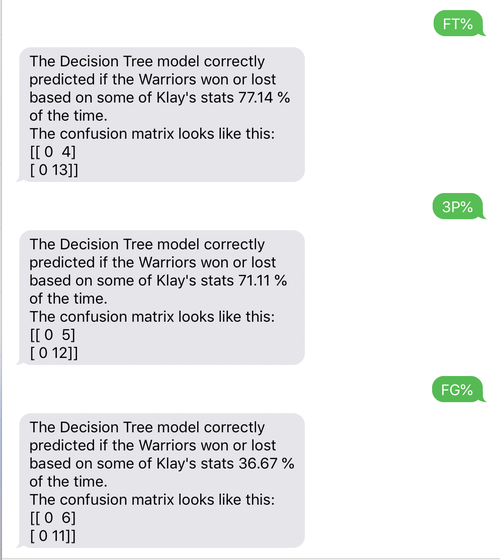The complete code can be found here on GitHub. It seems that Klay Thompson's free-throw percentage is better than three-point percentage in predicting whether or not the Warriors won that game, and field goal percentage is not good at predicting whether they won or lost

## What will you Predict Next?This is just the tip of the iceberg for developing with decision trees, Pandas, and Sci-kit Learn. You can also utilize specific algorithms like random forests and k-neighbors, make a Naive Bayes classifier, better optimize decision tree performance, use non-sports data, and more! Let me know in the comments or online what you're building with machine learning.

Authors
•Lizzie Siegle
Not ready yet? Talk to an expert.